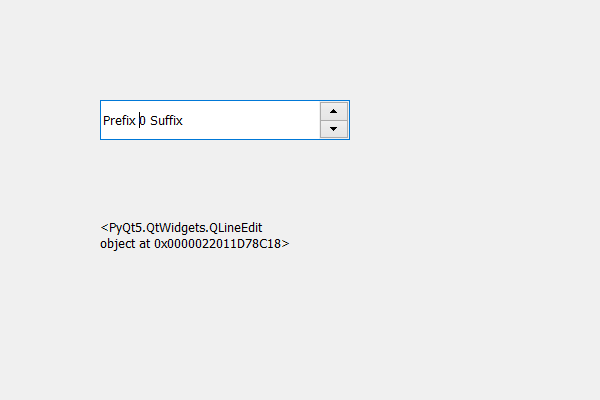# PyQt5 QSpinBox – Finding child through position

• Last Updated : 17 May, 2020

In this article we will see how we can get the child of the spin box at the given position inside the spin box, child are basically the component of the spin box, for example, the spin box main child is its QLineEdit which is used to see the value and set the value.

In order to do this we use `childAt` method.

Syntax : spin_box.childAt(x, y)

Argument : It takes position as argument it can be x, y co-ordinates or the QPoint object

Return : It returns child at given position if there is no child it return None

Note : The co-ordinates are not of the window co-ordinate system they are in spin box co-ordinate system

Below is the implementation

 `# importing libraries``from` `PyQt5.QtWidgets ``import` `*` `from` `PyQt5 ``import` `QtCore, QtGui``from` `PyQt5.QtGui ``import` `*` `from` `PyQt5.QtCore ``import` `*` `import` `sys`` ` ` ` `class` `Window(QMainWindow):`` ` `    ``def` `__init__(``self``):``        ``super``().__init__()`` ` `        ``# setting title``        ``self``.setWindowTitle(``"Python "``)`` ` `        ``# setting geometry``        ``self``.setGeometry(``100``, ``100``, ``600``, ``400``)`` ` `        ``# calling method``        ``self``.UiComponents()`` ` `        ``# showing all the widgets``        ``self``.show()`` ` `    ``# method for widgets``    ``def` `UiComponents(``self``):``        ``# creating spin box``        ``self``.spin ``=` `QSpinBox(``self``)`` ` `        ``# setting geometry to spin box``        ``self``.spin.setGeometry(``100``, ``100``, ``250``, ``40``)`` ` `        ``# setting prefix to spin``        ``self``.spin.setPrefix(``"Prefix "``)`` ` `        ``# setting suffix to spin``        ``self``.spin.setSuffix(``" Suffix"``)`` ` `        ``# creating a label``        ``self``.label ``=` `QLabel(``"Label "``, ``self``)`` ` `        ``# setting geometry to the label``        ``self``.label.setGeometry(``100``, ``200``, ``200``, ``70``)`` ` `        ``# getting the child at the point 10, 10``        ``child ``=` `self``.spin.childAt(``10``, ``10``)`` ` `        ``# setting text to the label``        ``self``.label.setText(``str``(child))`` ` `        ``# making label multi line``        ``self``.label.setWordWrap(``True``)`` ` ` ` ` ` `# create pyqt5 app``App ``=` `QApplication(sys.argv)`` ` `# create the instance of our Window``window ``=` `Window()`` ` `# start the app``sys.exit(App.``exec``())`

Output :My Personal Notes arrow_drop_up